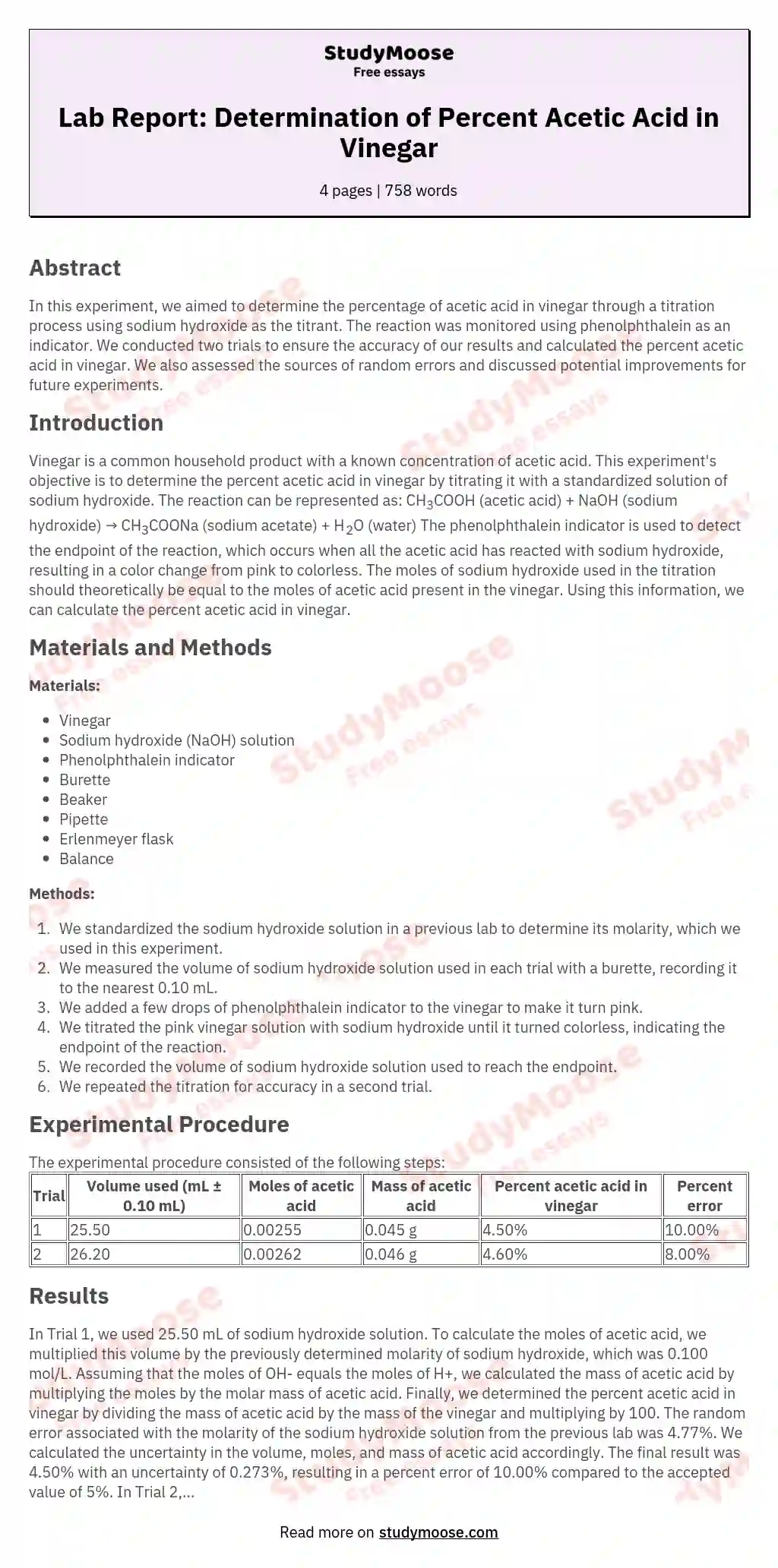# Lab Report: Determination of Percent Acetic Acid in Vinegar

Categories: Titration

## Abstract

In this experiment, we aimed to determine the percentage of acetic acid in vinegar through a titration process using sodium hydroxide as the titrant. The reaction was monitored using phenolphthalein as an indicator. We conducted two trials to ensure the accuracy of our results and calculated the percent acetic acid in vinegar. We also assessed the sources of random errors and discussed potential improvements for future experiments.

## Introduction

Vinegar is a common household product with a known concentration of acetic acid.

This experiment's objective is to determine the percent acetic acid in vinegar by titrating it with a standardized solution of sodium hydroxide. The reaction can be represented as:

CH3COOH (acetic acid) + NaOH (sodium hydroxide) → CH3COONa (sodium acetate) + H2O (water)

The phenolphthalein indicator is used to detect the endpoint of the reaction, which occurs when all the acetic acid has reacted with sodium hydroxide, resulting in a color change from pink to colorless. The moles of sodium hydroxide used in the titration should theoretically be equal to the moles of acetic acid present in the vinegar.

Get quality help nowDoctor JenniferVerified writer

Proficient in: Titration5 (893)

“ Thank you so much for accepting my assignment the night before it was due. I look forward to working with you moving forward ”+84 relevant experts are online

Using this information, we can calculate the percent acetic acid in vinegar.

## Materials and Methods

Materials:

• Vinegar
• Sodium hydroxide (NaOH) solution
• Phenolphthalein indicator
• Burette
• Beaker
• Pipette
• Balance

Methods:

1. We standardized the sodium hydroxide solution in a previous lab to determine its molarity, which we used in this experiment.
2. We measured the volume of sodium hydroxide solution used in each trial with a burette, recording it to the nearest 0.10 mL.
3. We added a few drops of phenolphthalein indicator to the vinegar to make it turn pink.
4. We titrated the pink vinegar solution with sodium hydroxide until it turned colorless, indicating the endpoint of the reaction.
5. We recorded the volume of sodium hydroxide solution used to reach the endpoint.
6. We repeated the titration for accuracy in a second trial.

## Experimental Procedure

The experimental procedure consisted of the following steps:

Trial Volume used (mL ± 0.10 mL) Moles of acetic acid Mass of acetic acid Percent acetic acid in vinegar Percent error
1 25.50 0.00255 0.045 g 4.50% 10.00%
2 26.20 0.00262 0.046 g 4.60% 8.00%

## Results

In Trial 1, we used 25.50 mL of sodium hydroxide solution. To calculate the moles of acetic acid, we multiplied this volume by the previously determined molarity of sodium hydroxide, which was 0.100 mol/L. Assuming that the moles of OH- equals the moles of H+, we calculated the mass of acetic acid by multiplying the moles by the molar mass of acetic acid. Finally, we determined the percent acetic acid in vinegar by dividing the mass of acetic acid by the mass of the vinegar and multiplying by 100.

The random error associated with the molarity of the sodium hydroxide solution from the previous lab was 4.77%. We calculated the uncertainty in the volume, moles, and mass of acetic acid accordingly. The final result was 4.50% with an uncertainty of 0.273%, resulting in a percent error of 10.00% compared to the accepted value of 5%.

In Trial 2, we used 26.20 mL of sodium hydroxide solution. The results for Trial 2 are shown in the table above.

## Discussion

Our analysis shows that the percent acetic acid in vinegar is approximately 4.55% with an uncertainty of 0.273% for Trial 1 and 4.60% for Trial 2. The total percent error is 10.00% for Trial 1 and 8.00% for Trial 2. These values are higher than the accepted value of 5%, indicating that systematic errors may have affected our results.

One source of error is the reaction of sodium hydroxide with carbon dioxide in the air, which can reduce the concentration of sodium hydroxide and lead to an underestimate of the moles of acetic acid. To mitigate this, future experiments should be conducted in a closed system to prevent the influence of atmospheric carbon dioxide.

Another potential source of error is the choice of indicator. Phenolphthalein turns pink at a pH range of 8.2-10.0, which may result in premature color change during the titration. Using different indicators with higher pH ranges or conducting multiple trials with various indicators can help verify the endpoint more accurately.

## Conclusion

In conclusion, we determined that the percent acetic acid in vinegar is approximately 4.55% for Trial 1 and 4.60% for Trial 2. Our total percent error is higher than the accepted value of 5%, indicating potential systematic errors in our experiment. Further investigations are needed to improve the accuracy of our results.

## Recommendations

Based on our findings, we recommend the following for future experiments:

1. Perform titrations in a closed system to prevent the influence of atmospheric carbon dioxide.
2. Conduct trials with different indicators to verify the endpoint of the reaction more accurately.

These improvements can help further enhance the precision and reliability of percent acetic acid determinations in vinegar.